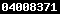### QUANTITATIVE RELATIONSHIPS BETWEEN MOLECULAR STRUCTURE AND MELTING POINT OF SEVERAL ORGANIC COMPOUNDShttps://doi.org/10.22146/ijc.21918

Iqmal Tahir(1*), Karna Wijaya(2), M Utoro Yahya(3), Muzakky Yapin(4)

(1) Austrian-Indonesian Centre for Computational Chemistry, Gadjah Mada University, Yogyakarta
(2) Austrian-Indonesian Centre for Computational Chemistry, Gadjah Mada University, Yogyakarta
(3) Austrian-Indonesian Centre for Computational Chemistry, Gadjah Mada University, Yogyakarta
(4) Akademi Perawatan Darul ‘Ulum, Jombang
(*) Corresponding Author

#### Abstract

Quantitative  Structure - Property  Relationships (QSPR) analysis of the Melting Point (m.p) of 349 organic compounds using molecular descriptors based on linear regression have been done. The experiment m.p. values were collected from the CRC Hand Book of Chemistry and Physic 81th . Molecular description of each compound was described in the form of the number of atoms and number of chemical bonding. This study was made in the form of normal melting point (in Kelvin unit) as the linear function of all molecular descriptor. The best equation model was determined by regression multilinear analysis and the result showed that the melting point is linearly correlated  with:   n(Csek alf), n(Cter alf), n(Csek sik), n(Cter sik), n(Csek arm), n(Cter arm), n(O), n(N), n(F), n(Cl), n(C-C sik), n(C=C arm), n(C1=C2), n(C2=C3), n(C-O), n(C-Br), n(C-I), n(N-H), n(Z), n(ortho), n(meta), n(para), (BM), n(C-H)2, n(H x BM) and n(Cprim x BM). The QSPR equation is significant at 95 % level and has statistical parameters: n = 349, r = 0.943, SD = 24.336 and Fexp = 99.326,  respectively.

Keywords: QSPR, melting point

#### Full Text:

Full Text PdfDOI: https://doi.org/10.22146/ijc.21918

#### Article MetricsAbstract views : 181 |views : 368

Copyright (c) 2010 Indonesian Journal of ChemistryView The Statistics of Indones. J. Chem.